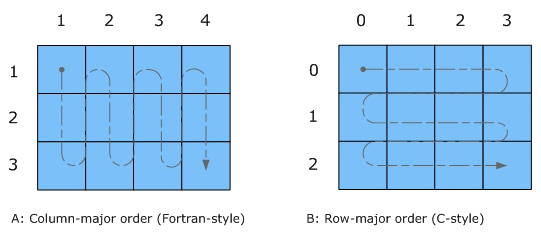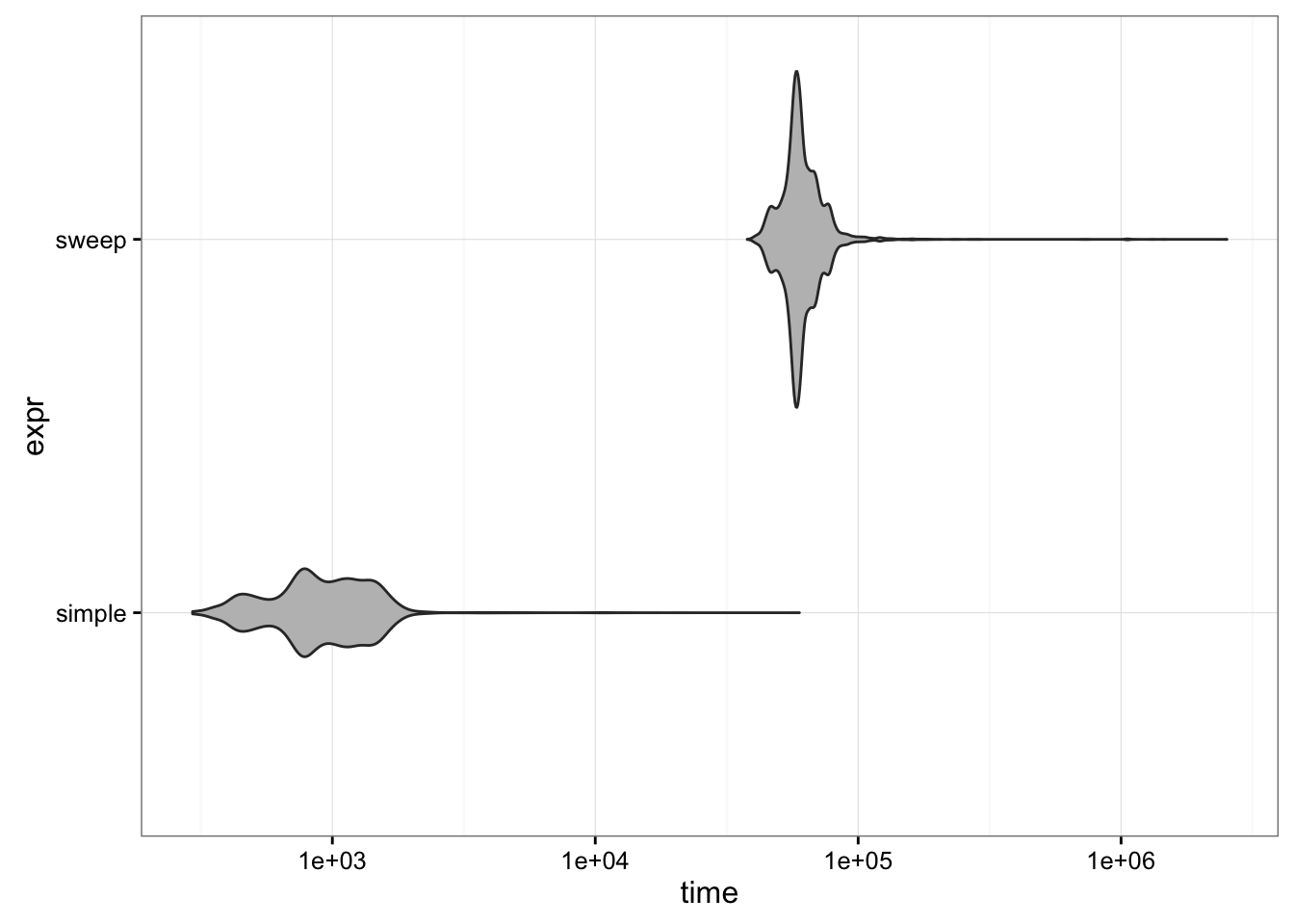Normalizing columns or rows of a data set is a very common operation. In particular, suppose you want to divide each row of a matrix by its sum, so that each row sums to 1.0. This would be sensible, e.g., if each column in the matrix represented a different species (OTU) and each row represented a sample, so that instead of counts the matrix represented sample proportions.

This is quick and easy in R by just dividing the matrix by its row sums:

m <- matrix(c(1:3,2*(1:3)),byrow=TRUE,ncol=3)
m/rowSums(m)
##           [,1]      [,2] [,3]
## [1,] 0.1666667 0.3333333  0.5
## [2,] 0.1666667 0.3333333  0.5

This is easy and fast, but works only because R happens to store matrices internally in column-major order: that is, underneath an R matrix is just a numeric vector (i.e., a one-dimensional string of numbers) that R knows should be treated as a matrix. If the matrix has $$r$$ rows, the first $$r$$ elements in the vector make up the first column; elements $$r+1$$ to $$2r$$ are the second column; and so forth.From Intel documentation

The second important ingredient is what R does when you ask it to divide one vector by another. If the vectors are different lengths, R automatically copies the shorter one until it is the right length. If the longer one is not an even multiple of the shorter one’s length, R gives a warning. In the case of dividing a matrix (i.e. a vector of length $$r \times c$$) by a vector of length $$r$$, this works seamlessly. R replicates the vector of row sums (length $$r$$) $$c$$ times; because R stores matrices in column-major order, the replicated vector of row sums lines up correctly with the matrix. However, if you innocently asked for cs <- colSums(m); m/cs, you would get gibberish, without a warning: R would replicate the length-$$c$$ vector $$r$$ times and divide. In our example above, the first element m[1,1] would be divided by the sum of the column 1 (cs): so far, so good. But R would next take m[2,1] (second row, first element) and divide it by cs, m[1,2]/cs, m[2,2]/cs, and so forth … ugh.

The more explicit, and therefore safer, way to scale the rows by their row sums is to use the sweep() function:

sweep(m,MARGIN=1,FUN="/",STATS=rowSums(m))
##           [,1]      [,2] [,3]
## [1,] 0.1666667 0.3333333  0.5
## [2,] 0.1666667 0.3333333  0.5
• MARGIN means you want to operate on rows (rows=1, columns=2; this is just something you have to remember)
• FUN is the function you want to apply to each piece of the matrix. Here we want to scale by dividing, but sometimes we might want to multiply, subtract, etc.
• STATS is the vector we want to “sweep out” (in this case, divide by)

Now if we wanted to switch to scaling columns instead of rows we would just use MARGIN=2 and STATS=colSums(m), whereas if we wanted to use division we would have to be very tricky (t(t(m)/colSums(m)))).

Division is about 100x times faster than sweep() - but unless you have a truly gigantic data set or need to do this operation millions of times, it probably won’t matter: